Next: Conclusions to Chapter 1 Up: Kinematics of special relativity theory Previous: The relativistic law for velocity addition   Contents

# Additional criticism of relativistic kinematics

We shall begin with some general remarks. The group properties of mathematical equations, as the transformations with mathematical symbols, do not bear any relation to any physical principles or postulates; that is, the group properties can be found without additional physical hypotheses. For example, the Lorentz transformation laws, which reflect the group properties of the Maxwell equations in vacuum (or of the classical wave equation, including that in the acoustics), are not bound at all with SRT's postulate of constancy of the speed of light or with the relativity principle.

The theory of relativity is, in fact, "the theory of visibility": it is about what we see in an experiment, if it is based (with generalization for space and time properties) on the laws of electromagnetic interactions (the absolutisation of electromagnetic phenomena). Similarly, the question can be raised: What will the phenomena observed by means of sound, etc., look like? Certainly, the finiteness of the rate of transmission of some interactions alters the phenomena observed with the help of these interactions. But this circumstance does not prevent making unique extrapolations for "binding" to space and time (which are the absolute physical notions) for the unique description of the world without limitation by any "overall" hypotheses.

Newtonian space possesses an important property: systems with lower dimensions can possess similar properties. For example, a vector can be introduced not only in three-space, but also on a plane and on a straight line. In RT, three-dimensional quantities do not possess vector properties (only the 4-vectors do this); that is, there is no continuous limiting transition to classical quantities (the "nearly vector"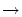vector).

As the next remark, we shall describe the "non-locality" paradox. Note that all SRT formulas do not depend on the previous history of motion, i.e. they are local. Let system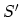move at velocityrelative to system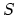. Let a light flash occur at centerat the time of its coincidence with center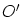. At time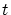in system, let the wave front reach point, and in system- point, respectively (Fig. 1.24).Now we impart, by pulse, velocityto a signal receiver in systemat point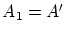. It happened that the wave front has moved right away to(since we are now in system). But where had the wave front been at the same time instant? Did the time atchange? And if we will stop the receiver at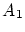after a moment? The time will be restored, and the wave front will again return to? And the observer will forget that he saw a flash of light? Then, in order to see the future, one must move faster? The fact that the observer athad not at all times moved together with systemexplains nothing, since another observer, who had all the time moved together with system, could be at. Does it occur that one of them will see the event, whereas the other one will not? If so, the objective nature of science disappears.

The next additional issue is as follows. Does a wave packet (light) move in vacuum at light speed? If yes, then we cannot break it down into (separate) pulses (signals) by means of a stroboscope: due to length shortening, the length of each pulse and the length of each interval between the pulses must be zero (which is contradictory). If, however, we suppose the dimensions of obtained pulses (signals) and intervals between them to be finite in the resting (laboratory) coordinate system, then in the intrinsic reference system of package, both pulses and intervals should be infinite (but how can we interrelate in this case the pulse and the interval, where it is absent?). In essence, it is the following question: whether light and the space between signals are material or not?

Let us make now some comment about a change of the visible direction of particle motion or about a change of the visible direction of wave signal arrival (remember the aberration, for example) as an observer goes to other moving system. This simple classical fact is described in SRT as the turn of all wave front at some angle. As this takes place, the wave front presents a light sphere at the same time instant. We would remind that the wave front in SRT is different at the same time instant for systems moving relative each other (just as the result of a change in running of time). However, the prehistory of motion of recording instruments is included in none SRT formula. If a photon has been flying in space between a source and a receiver, it is causally connected in no way with motion of the source and the receiver at the same time instant. The interaction of the recording instrument with the photon occurs just at the time of signal reception only. No difference exists whether the receiver had been having a velocityall the time and was brought into this space point at the time of signal reception, or it had been being motionless at the same space point, but acquired the same velocityat the instant before signal reception (the result of interaction with the photon were the same in both cases). Thus, the only fact of photon arrival to the given place of space matters for the fact of receiving of a photon as such. Obviously, the value of some velocity at the given place of space does not change the fact of signal arrival as such (but, according to the Doppler effect, its frequency will be changed only). If the fact itself of the signal receipt were dependent on this, then what does the substitution of values in the Doppler formula at the one of observation systems mean? Therefore, no real turn of all wave front can be (since it reflects the fact of signal arrival). This is the local (at the given point) mathematical (differential) method to determine the visible direction of signal reception. It can be easily understood by analogy with the usual natural phenomena - with rain and snow (Fig. 1.25).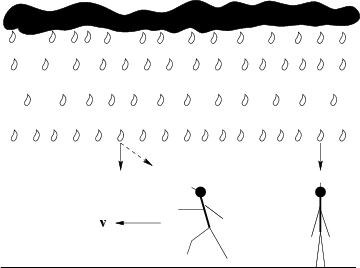If you look at a cloud over your head in the windless weather when it is raining, you see vertical fall of drops (the direction of "signal" reception). But if you will run (it is better to remember a car travel in a snow day), the direction of drops arrival (the direction of "signal" reception) will far ahead along motion and can be lack of coincidence with the real cloud. However, the horizontal front of rain either already reached the earth surface (the fact of "signal" reception), or not, and this fact does not depend on your motion at the given point of the earth surface at all (see Fig. 1.25).

Let us discuss some speculative constructions of SRT. So, unreal in SRT is the consideration of infinite systems, such as a conductor with current, in "explaining" the appearance of additional volume charge (the game with infinities). In reality, the conductor can be close-loop (finite) only. In this case the explanation is not only complicated methodically, but also contradictory. Let us consider a square loop with current (for example, a superconducting loop). The value of a charge of each electron and ion is invariant; the total number of particles is invariable too. How can change the density of charges in this case? Consider the motion of electrons from the viewpoint of a "system of ionic grid" (Fig. 1.26).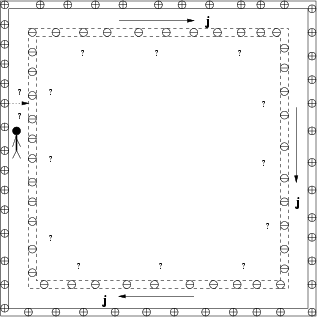According to SRT, the "electronic loop" should decrease in size (the contraction of lengths because of motion of electrons on each rectilinear section). It would seem that, owing to symmetry of the problem, the "electronic loop" should enter inside the "ionic loop". However, in such a case we would have a strange asymmetrical field (of dipole type) near the conductor. Besides, while moving at high velocity, the electrons and ions could appear on different sides from the observer. It is completely unclear, how such a transition through the observer (perpendicular to the motion of particles!) could take place at all? And by what forces the charged electrons (as well as the ions) would be retained together in a flux, not flying away to different sides? Even if we take advantage of the fitting SRT uncertainty (towards what end does the contraction occur?) for one side of a square, all questions still remain for its other sides.

The SRT's system of watches and rules is inconvenient both theoretically and practically, since it supposes that all the data are gathered and analyzed (interpreted!) somewhat later. The uniqueness of interrelation between the classical Newtonian and relativistic Lorentzian coordinates does not imply automatic consistency of latter ones (just in this, physical sense consists the distinction of physics from mathematics). For example, we could use in all SRT formulas the speed of sound in air instead of speed of light and consider the motions on the Earth at subsonic velocities in resting air. However, the inconsistency of similar transformations (for the time) would be immediately revealed in the experiment. This fact demonstrates the hazard of formally mathematical analogies for physics.

It is obvious that the relativistic hypothesis for time dilation is wrong, since only the square of relative velocity is included in the formula (the effect does not depend on the velocity direction). Take 4 identical objects. Let second object be moving at some velocity V1 relative to the first one, then its time will be slowed down relative to the time of the first object. You say that it is an objective effect? (We would remind the meaning of the word "objective": an effect does not depend on presence and properties of the observer which not interacts with the object under study.) We even would not fly to check it. Let the third object be moving relative to the second one in an arbitrary direction with some velocity V2. Then, by analogy, its time will be slowed down relative to the time of the second object. Is this effect objective again? Let the fourth object be motionlessly placed near the first object. We even does not try to debate with which velocity the fourth object moves relative to the third object: it is important only that in the general case this velocity does not zero. Therefore, again we have "objective relativistic" time dilatation of the fourth object relative to the third one. Thus, dt1 > dt2 > dt3 > dt4. But dt1 = dt4 (and we have no need to fly somewhere), since the fourth and first objects are in relative rest! Similar absurdity was obtained as the result of a fanatic relativistic faith in the uniqueness and infallibility of Einstein's method of synchronization in pairs. Objectiveness melt away from under feet, and a remainder is either the relativistic seeming effect or pure rated combinations ("floating time belts"). What matter is the declared greatness?

Now we shall make some general remarks. The whole SRT kinematics follows from the invariance of the interval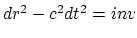. However, we see that this expression is written for the empty space. In a medium the speed of light is non-constant, it can be anisotropic, and the light of non-arbitrary frequency can propagate in the given particular medium (remind the attenuation, absorption, reflection, dissipation). There is no sections of physics, where the properties of phenomena in vacuum would be automatically transferred to the phenomena in other media (for example, in liquids - hydrodynamic and other properties; in solid bodies - elastic, electrical and other properties). That is, they are not determined by the properties of the empty space. And only SRT pretends to a similar universal "cloning" of properties.

Generally speaking, the properties of light, which are intrinsically contradictory and mutually exclusive, are simply postulated in SRT. Therefore, wrong is Fock's  statement, that the light is a simpler phenomenon, than the rule. It is not worth to extol the role of light signals and all "visible things"; otherwise a teaspoon inside a glass with water could be considered as the broken one (pure geometrically, the fallacy in this consideration can easily be tested by the direct location of coordinates of all "teaspoon outlets" at the boundary of the liquid). The classical time (or the time determined by an infinitely remote source at the middle perpendicular to the line of motion) possesses some important advantage: we know a priory that it is identical everywhere, and no calculations or discussions are required concerning the prehistory of the process or properties of the space. Actually, SRT uses the speed of light as one of measurement standards. Remind that in the classical kinematics there are two measurement standards: the length and time (we will "formulate" evident "laws of constancy of standards": the length of the standard of 1 m is constant and is equal to one meter, the duration of the standard of 1 sec is constant and is equal to one second, but relativists din "the Great Law of Constancy of the relativistic standard" into everyone's ears). Since the introduction of a standard is the definition, its properties are not subject to discussion . As a result, everything, which is related with the light propagation, ceases to be a prerogative of experiment in SRT. And because all derivations in SRT are written only for the events - the light flashes, then SRT occurs to be logically inconsistent (to say nothing of the fact, that the "use" of properties of light in vacuum is profusely spread to all other "non-vacuum" phenomena).

Feynman in his book  says with sarcasm about the philosophers and about the dependence of results on the frame of reference, but he does not emphasize that, in spite of any "appareness", the subjects have real objective characteristics. For example, a man may seem to have a size of ant from the great distance, but this does not mean that he has really reduced (all instruments are used to be calibrated just under objective characteristics). The reasoning on a relativity of all quantities seems to be realistic, but (!) once the time in SRT became relative and the rate of interaction was supposed to be finite, the notion of relative quantity for spatially separated objects has become indefinite (It depends on the path of connection, is not bound causally, depends on the system of observation, etc). The definition of all quantities with respect to "far stars" is senseless, since we can see a "never existing reality". For example, the Alpha-Centaur has been at this particular place and possessed such properties 4 years ago; the other stars have been the same as we see tens or hundreds years ago and the distant Galaxies - billions years ago, i.e. the signal was sent when the earth observer did not exist yet, and is accepted when, possibly, the source itself no longer exists. In such a case, relative to which should we determine the quantities? It is clear that the relative quantities can be determined only with respect to the local characteristics of space (the unique instantaneous causal bond).

Some important remark concerns the notion of relativity, which has even entered the name of the SRT theory. Contrary to Galileo's ideas on isolated systems, an interchange of light signals between systems is used in SRT. The notion of relativity has been worked up to nonsense in SRT and lost its physical sense: in fact, the system with several (as a rule, two) objects is singled out, and the whole remaining real Universe is eliminated. If such an abstraction can even be postulated in SRT, then, the more so as, one can simply postulate the independence of processes inside the separated system on the velocity of system motion relative to the "emptiness" which remained from the whole Universe. But, even in spite of such an abstraction, no "real" relative quantities will appear for bodies (such as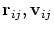, etc.). Indeed, the response of bodyto the attempt of changing its state is determined by the local characteristics: the state of a bodyand the state of the fields at the given point of space. But the changes having occurred with bodywill have an effect on the other bodies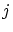only in some time intervals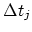. Thus, all changes of quantities should be determined relative to the local place (or local characteristics). And these phenomena just represent manifestations of the Newtonian absolute space. The question, whether the separated direction and separated coordinate origin (either moving or resting) exist in this absolute space - is quite different question. In the abstract (model) theories this question can be postulated, for example, from the considerations of convenience of the theory; but for our unique real Universe it should be solved experimentally. The absolute time notion in the classical Newtonian physics was extremely clear as well. The time should be uniform and independent of any phenomena observed in a system. Exactly such a property is inherent in the time synchronized by an infinitely remote periodic source on a middle perpendicular. However, in SRT the time is not an independent quantity: it is associated with the state of motion of a system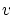and with the coordinates, for example, by the relation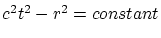. For uniform running of time the choice of the time reference point is arbitrary. For unified description of the phenomena and for comparability of the results the scales (units of measurement) should be identical for all systems. The time running uniformity automatically ensures the greatest simplicity of description of the phenomena and for the basis notion of time allows to introduce its standard definition.

Let us make some more methodical comments. Generally speaking, in SRT the method of comparison of the phenomena in two various inertial systems supposes, that both these systems have existed for infinitely long time. However, the systems have often been "linked" to particular bodies and have existed for a finite time only. Then, in each particular case the question needs to be studied: whether the prehistory of formation of these systems (its influence) has been "erased" or not?

The Euclidean analogies with projections in the book  are completely inadequate to the reality. The projection is only an abstract method of description, the subject itself does not change at turning. In SRT, on the contrary, the characteristics of an (even remote) object instantaneously change with changing the motion of an observer (!).

The limiting transition from the Lorentz transformations to the Galileo transformations (for the time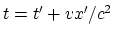) indicates that the Newtonian mechanics is not simply a limit of low velocities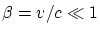, but the other condition is required, namely: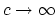. But in this case for many quantities in SRT there is no limiting transitions to classical quantities (see below, or ). However, in the classical physics: its finite value was measured even in 17th century!

The property of maximum homogeneity of the space-time can be an attribute of either ideal Newtonian mathematical space and time (being actually a "superstructure from above"), or of the model space (for example, with remotely non-interacting material points). The attempt to rest upon the mentioned property in RT as on the principal property of the real space and time is artificial. First, even in the earth scales we can not arbitrarily change the points of space, time instants, directions of axes and velocities of inertial systems (recall the limited nature of the Earth space, the rotation of the Earth, the gravitational field, the effect of the Moon, the electric, magnetic, temperature fields and so on). We have listed above the real achieved practical limitations, rather than the principal restrictions somewhere at relativistic velocities and huge scales of the Universe. True, in the scales of the Universe with its real objects and gravitational fields this property is not confirmed too: the model of uniform "jelly" does not describe the real Universe. Second, in addition to the form of equations, the solution is still determined mathematically by the boundary and initial conditions. This also actually, on real finite scales, prevents any shifts and changes (or it is necessary to change, in addition, the imposed conditions). How can we approach the existing nonlinear properties and equations with the RT claims? Even the "relativity" notion itself does not allow us to generalize (more likely, to narrow down) the real space with gravity. (As Fock  has emphasized, the "general relativity theory" term is inadequate).

The principle of relativity (in any form) supposes that "without looking" outside the limits of a system it is impossible to discover its uniform motion. Earlier it was the ether, which has played a part of the all-penetrating medium for possible discovering such a motion. Note that the question was not about the discovery of the absolute motion, but only about the motion relative to ether. That is, it would be possible to compare these motions "without looking" outside (here we keep in mind the calculating possibility only, since the system of registration points and standards cannot be tied with the ether). But even with "canceling" the ether, according to the modern concepts, still remains the "candidate" with similar properties - the gravitational field (which is principally non-shielded). For example, from the relic radiation anisotropy, under the additional hypothesis on the equality of the rate of propagation of gravitational interactions and speed of light, may follow the anisotropy of the (all-penetrating) gravitational field. Thus, the non-equal rights of inertial systems in macroscales can be found, in principle, "without looking" outside even at the local point. This can be avoided theoretically under the hypothesis, that the rate of propagation of gravitational interactions is much higher than the speed of light; in such a case the isotropy could be set up, but in actual practice - it is the prerogative of the Experiment.Next: Conclusions to Chapter 1 Up: Kinematics of special relativity theory Previous: The relativistic law for velocity addition   Contents
Sergey N. Arteha